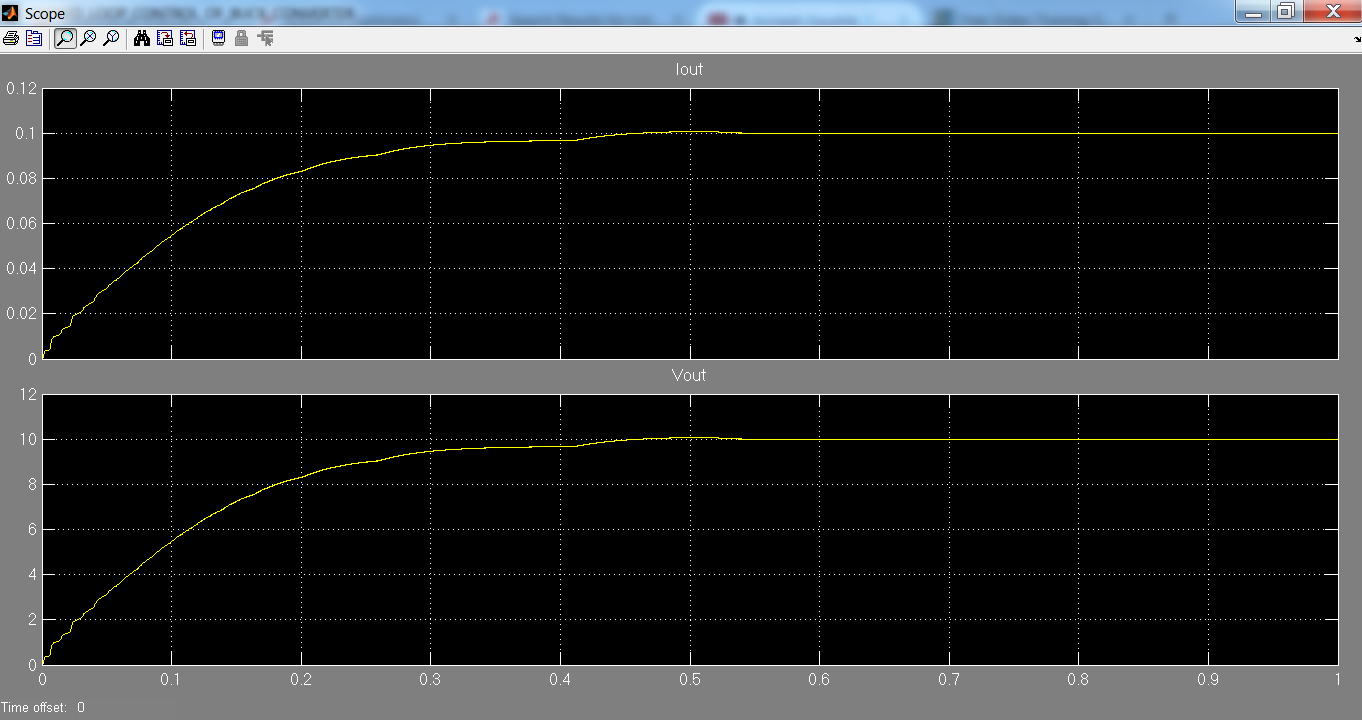## Closed Loop Control of Buck Converter

Call for Price

Buck Converter is a converter which converts variable DC voltage to required DC voltage. Buck converter is used to get DC output voltage lesser than the input voltage. Closed loop control is a process by which the output voltage is maintained constantly by obtaining the feedback of the loop.

SKU: Closed Loop Control of Buck Converter-MiniProject Category:

## Introduction to Closed Loop Control of Buck Converter

Buck Converter is a converter which converts variable DC voltage to required DC voltage. Buck converter is used to get DC output voltage lesser than the input voltage. Closed loop control is a process by which the output voltage is maintained constantly by obtaining the feedback of the loop..

Closed loop control of Buck Converter is used to obtain a constant DC output voltage. The switching frequency and duty cycle determines the output voltage. In the closed loop process the output voltage is compared with a set voltage and the error value is reduced by controlling the switching pulse. The basic operation is if the error value is positive the duty cycle is reduced and if the error value is negative the duty cycle is increased by continuing the process continuously the output voltage is maintained constant.

## Matlab Simulink Block Diagram for Closed Loop Control of Buck ConverterThe above Matlab section explains the closed loop control of Buck converter. The output voltage is controlled with the help of a switching frequency applied to the switching device.

## Output waveform of Closed Loop Control of Buck Converter